# Solved How to select an Edge

Hi,
I know how to select a point / face, but I still don't know how to select an edge. Does each edge have a unique ID? For example, two quadrilateral faces have seven edges. If I choose an edge, I can know that its ID is 6.
I have a picture below. I have marked the edges I want to choose, but I don't know what to do. Can have a simple code demonstration?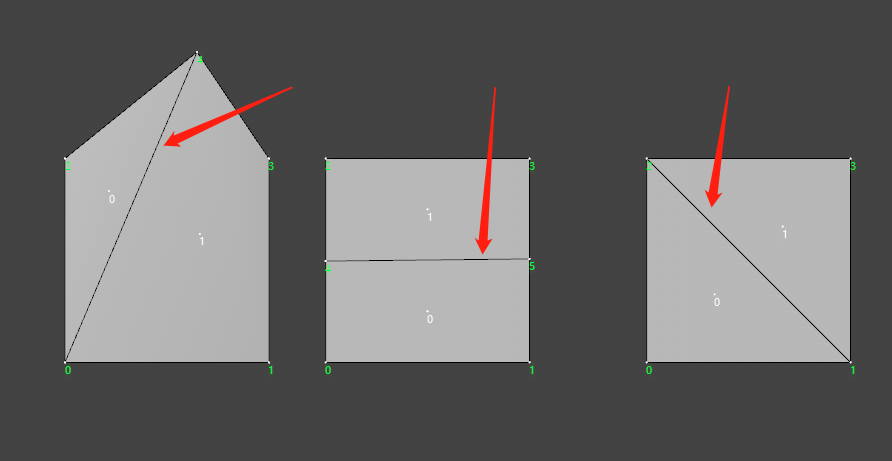Thanks for any help!

Hi @cairyn ,thanks for your help!
I have made some progress, but there are still some problems!

the image_1 show want wo select 2 edges.
image_1: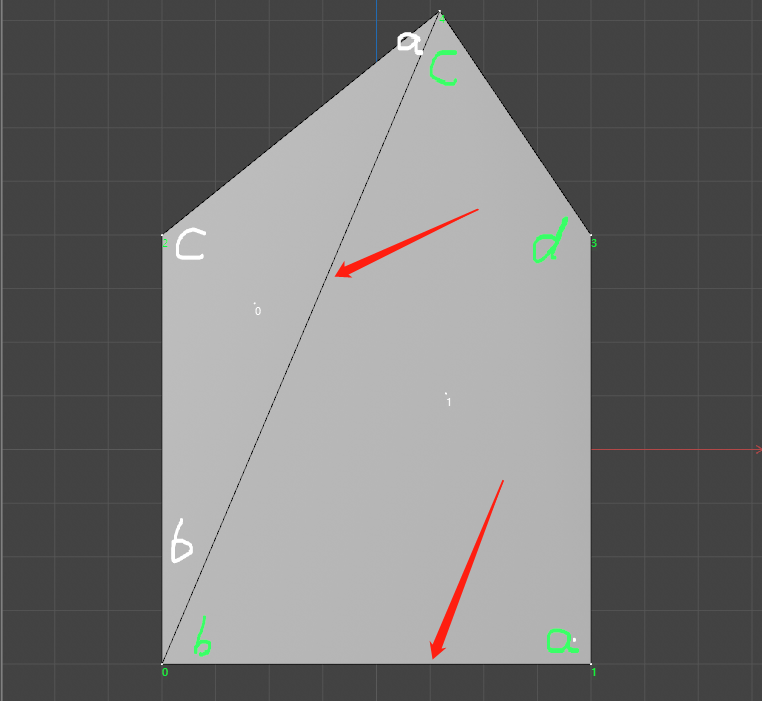Through your reply, it seems that I have got 2 directions to solve my problem. I will show it in the following image_2.
image_2: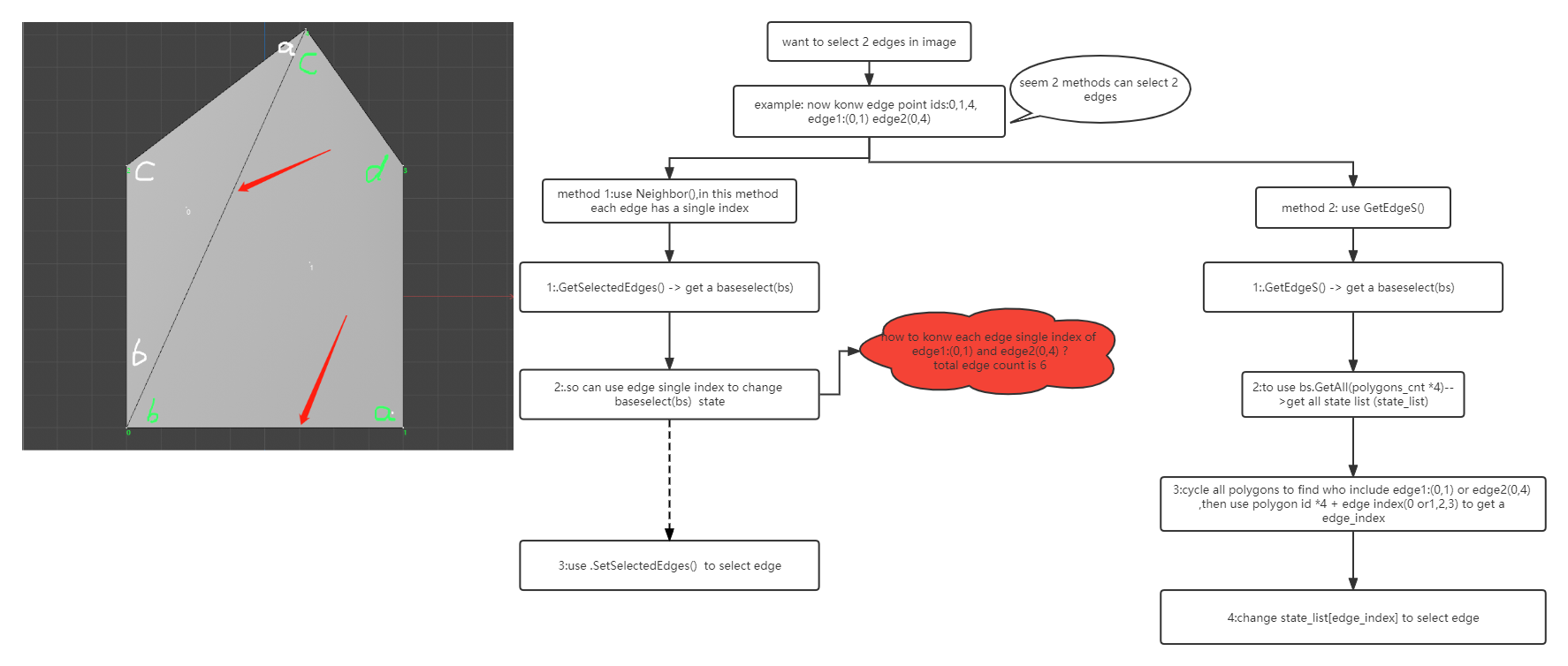use method 2 ,can select the edge show on gif_1.
gif_1: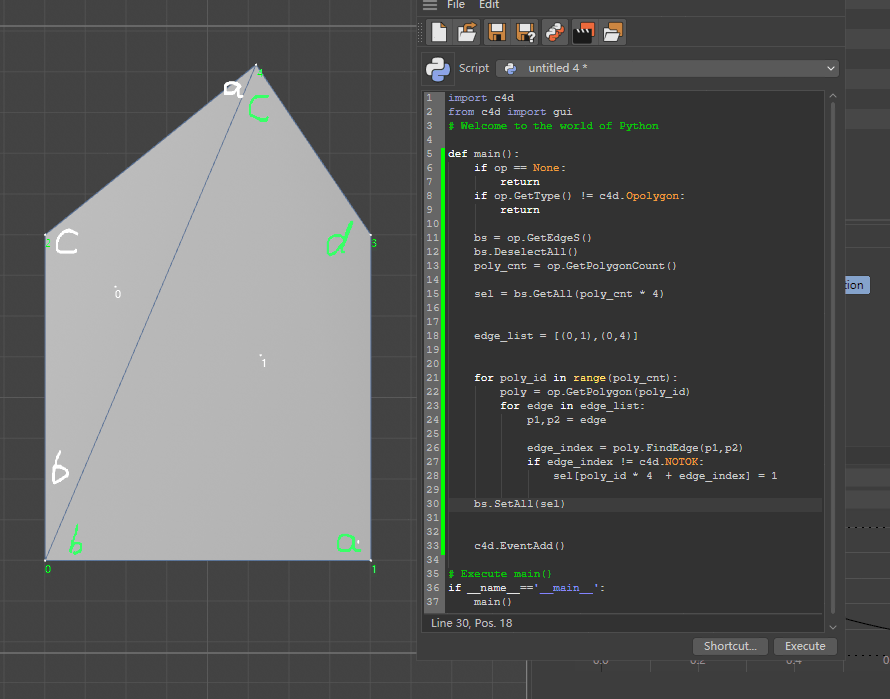method 2 code:

``````import c4d
from c4d import gui

def main():
if op == None:
return
if op.GetType() != c4d.Opolygon:
return

bs = op.GetEdgeS()
bs.DeselectAll()
poly_cnt = op.GetPolygonCount()

sel = bs.GetAll(poly_cnt * 4)

edge_list = [(0,1),(0,4)]

for poly_id in range(poly_cnt):
poly = op.GetPolygon(poly_id)
for edge in edge_list:
p1,p2 = edge

edge_index = poly.FindEdge(p1,p2)
if edge_index != c4d.NOTOK:
sel[poly_id * 4  + edge_index] = 1

bs.SetAll(sel)

# Execute main()
if __name__=='__main__':
main()
``````

While points and polygons are objects in C4D that are identified by an index of an array (where the actual point/polygon data is stored), edges are not - they exist only as "inferred" data and will be described through the polygons they belong to, and an "edge index" within that polygon.

If you look at the `PolygonObject` and the method `GetEdgeS()`, you will find the text:
"The edges are indexed by 4 * polygon + edge where polygon is the polygon index and edge is the edge index between 0 and 3."
(The docs don't tell, but it's kinda obvious: "edge index" 0 is between points a and b of the polygon, 1 between points b and c, 2 between points c and d, and 3 between points d and a.)
This will result in an "encoded enumeration" (or an ID, if you want) for the edge. (But it's not an index in the sense of points and polygons, as there is no edge data that this index could point to.)

Now, if you look at the first example and want to know what that edge's "ID" is, you may ask: Is that encoded through polygon 0 or polygon 1? Well, both. The simple code fragment

``````    bs = op.GetEdgeS()
print(bs.GetCount())
``````

will result in the count 2 if you just select the middle edge.

Looking at the full edge selection data:

``````import c4d
from c4d import gui

def IterateSelected(selection, total):
segments = selection.GetSegments()
for seg in range(0, segments):
minS, maxS = selection.GetRange(seg, total)
for index in range(minS, maxS+1):
yield index

def PrintSelectedEdgeIndex(obj):
if op == None: return
if op.GetType() != c4d.Opolygon: return

total = op.GetPolygonCount() * 4
selection = op.GetEdgeS()
print ("Selected Edges:")
for index in IterateSelected(selection, total):
print ("Polygon:", index // 4, "Edge:", index % 4)

def main():
if op == None: return
if op.GetType() != c4d.Opolygon: return

PrintSelectedEdgeIndex(op)

if __name__=='__main__':
main()
``````

you will get

``````Selected Edges:
Polygon: 0 Edge: 0
Polygon: 1 Edge: 1
>>>
``````

(well, since you didn't write the ABCD components of the polygons into your diagram, this is assuming that the polygons are 402- and 1043.) As you may guess, if you change the sequence of points in the polygon by "Change Point Order", the "ID" of the edge changes with it!

So, an edge has as many "IDs" as there are polygons which share it. This requires care if you want to change the edge selection because you must ensure that all those "IDs" are selected, otherwise you get an inconsistent selection.

If you go through the methods of a PolygonObject, you will find the additional ones: GetSelectedEdges and SetSelectedEdges, which make use of the `Neighbor` object. There, edges only have one "ID". I'm leaving you with the official documentation for this class though, since there are many examples and diagrams to see:
https://developers.maxon.net/docs/Cinema4DPythonSDK/html/modules/c4d.utils/Neighbor/index.html#c4d.utils.Neighbor

Hi @cairyn ,thanks for your help!
I have made some progress, but there are still some problems!

the image_1 show want wo select 2 edges.
image_1:Through your reply, it seems that I have got 2 directions to solve my problem. I will show it in the following image_2.
image_2:use method 2 ,can select the edge show on gif_1.
gif_1:method 2 code:

``````import c4d
from c4d import gui

def main():
if op == None:
return
if op.GetType() != c4d.Opolygon:
return

bs = op.GetEdgeS()
bs.DeselectAll()
poly_cnt = op.GetPolygonCount()

sel = bs.GetAll(poly_cnt * 4)

edge_list = [(0,1),(0,4)]

for poly_id in range(poly_cnt):
poly = op.GetPolygon(poly_id)
for edge in edge_list:
p1,p2 = edge

edge_index = poly.FindEdge(p1,p2)
if edge_index != c4d.NOTOK:
sel[poly_id * 4  + edge_index] = 1

bs.SetAll(sel)

# Execute main()
if __name__=='__main__':
main()
``````

Hi... not sure whether you see a problem in method 2, it seems fine to me (works, too).
(Specifically, it works because FindEdge does not care about the direction of the edge, otherwise the middle edge might give you trouble because it runs 4-0 for poly 0, and 0-4 for poly 1. But you do not need to consider the directionality/normal of the polygon here.)
(Also, border edges are only found once because there is only one polygon they belong to, but that is kinda obvious.)

Now, the biggest issue seems to me that the algorithm needs to find the polygons the edges belong to. AFAIK the PolygonObject does not carry much permanent relationship data between points, polygons, and edges; only the polygon references its point indices. Everything else needs to be found by an existing method or by walking through the whole structure.

The Neighbor class however must be initialized before usage, so in terms of speed, I wonder what is faster... Neighbor may internally initialize structures that you never use, but it is written in C++ so perhaps faster. Addressing the polygon object structure directly would be Python code, so slower, but more targeted. In case of large poly counts this may make a difference... I guess a Maxon person would be able to answer that. (I could measure runtimes directly and investigate, but I don't have the leisure to.)

Let me just look whether I have a fitting example for `Neighbor`...

Okay, I cobbled together an example for Neighbor, but I found the class more disappointing that I remembered it.
Neighbor doesn't seem to have many functions relating to the internal edge indices: notably, there is no method that returns the edge index for its two end points. This would make the algorithm very short (just look up all the neighbor edge indices for the given point pairs and create a BaseSelect from them).

Instead, you can only use GetEdgePolys to find the (at most) two polygons bordering the edge given by two points. This saves you the explicit loop over all polygons... but from there, you still need to use GetPolygon to retrieve the CPolygon from the indices, then FindEdge to get the poly's edge index, then GetPolyInfo to finally get the edge's neighbor-internal index that you can use in the BaseSelect.

It works, but unfortunately I am not convinced that it is faster or easier to read than method 2. meh

``````import c4d
from c4d import gui

def IterateSelected(selection, total):
segments = selection.GetSegments()
for seg in range(0, segments):
minS, maxS = selection.GetRange(seg, total)
for index in range(minS, maxS+1):
yield index

def PrintSelectedEdgeIndex(bs, total):
print ("Selected Edges:")
for index in IterateSelected(bs, total):
print ("Neighbor Edge:", index)

def main():
if op == None: return
if op.GetType() != c4d.Opolygon: return
ng = c4d.utils.Neighbor()
ng.Init(op)
print("# of edges:", ng.GetEdgeCount())

# show currently selected edges
bs = op.GetSelectedEdges(ng, c4d.EDGESELECTIONTYPE_SELECTION)
total = op.GetPolygonCount() * 4
PrintSelectedEdgeIndex(bs, total)

# edge list as input for the selection
edge_list = [(3,0),(0,4)]

bsNew = c4d.BaseSelect()
for edge in edge_list:
p1,p2 = edge

for poly in ng.GetEdgePolys(p1, p2):
# print ("Poly:", poly)
if poly != -1:
pinf = ng.GetPolyInfo(poly)
edge_index = op.GetPolygon(poly).FindEdge(p1,p2)
if edge_index != c4d.NOTOK:
ng_edge_index = pinf["edge"][edge_index]
print ("Poly:", poly,
"Edge index:", edge_index,
"Neighbor edge index:", ng_edge_index)
bsNew.Select(ng_edge_index)

op.SetSelectedEdges(ng, bsNew, c4d.EDGESELECTIONTYPE_SELECTION)

if __name__=='__main__':
main()
``````

(Also, I didn't catch any errors, and the code is not optimized in any way.)

Hi @Cairyn
Thanks for your help, I use method 2 to achieve my goal.
This is a plug-in to mirror a selection of the same / different objects. Thank you for your help again,gif_2 show its work
gif_2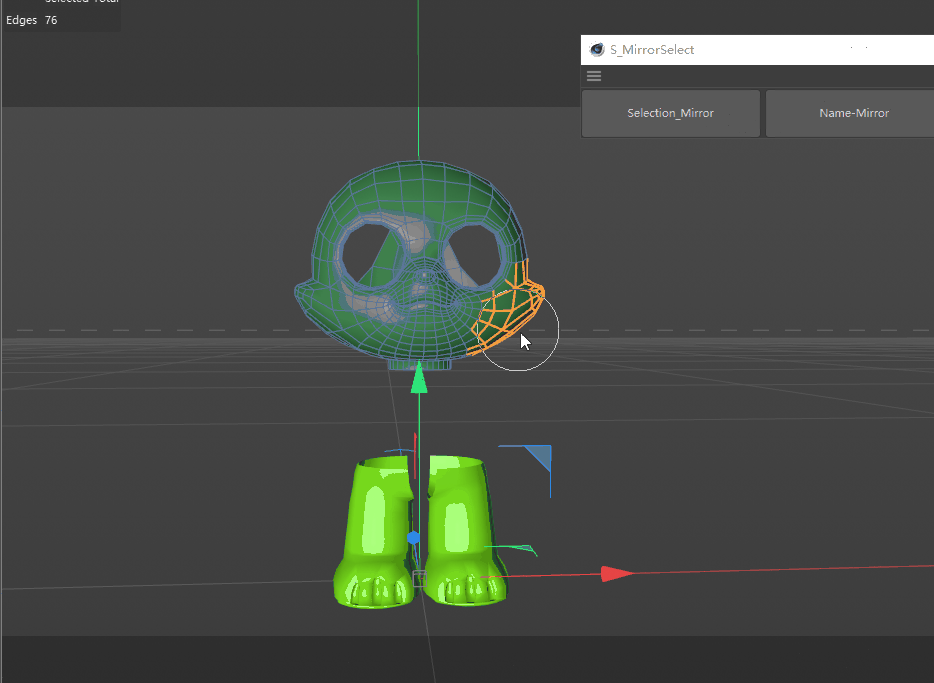Hello @chuanzhen,

thank you for reaching out to us. And thank you @Cairyn for providing an answer. We do not have anything to addd here.

Cheers,
Ferdinand

MAXON SDK Specialist
developers.maxon.net